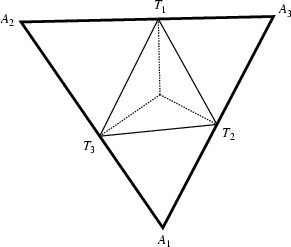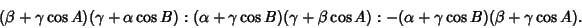## Antipedal TriangleThe antipedal triangleof a given Triangleis the Triangle of whichis the Pedal Triangle. For a Triangle with Trilinear Coordinatesand Angles,, and, the antipedal triangle has Vertices with Trilinear CoordinatesThe Isogonal Conjugate of the Antipedal Triangle of a given Triangle is Homothetic with the original Triangle. Furthermore, the Product of their Areas equals the Square of the Area of the original Triangle (Gallatly 1913).